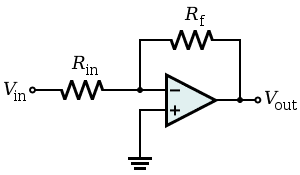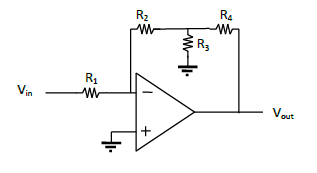Simple Op-Amp Inverting Amplifier

Homework Statement

I need to find an expression for the gain in this circuit. I know it is an interting amplifier, because with the models we were given to look at, this one fits the inverting amlpifier. I've also constructed a simulation in a simple Java applet that I have.

My problem is, I need to do this analytically. It is very late right now and I know I'm just having a brain fart. This is what the simplest inverting amplifier looks like:It's fairly obvious that, given a little thinking, the gain on this circuit:

$\frac{V_{in}}{R_{in}} = \frac{-V_{out}}{R_{f}} \rightarrow V_{out} = \frac{R_{f}}{R_{in}} V_{in}$

The problem is, with my circuit, Rf isn't just one resistor. It's 3, in a very strange configuration, and I cannot for the life of me figure out how to find the total resistance in that part of the circuit. This is my circuit:I tried looking at it as sort of a voltage divider, but that third resistor connected to ground is really screwing with my head! Help!

Homework Equations

V = IR is basically all I think you'd need.

The Attempt at a Solution

See above description.